## Example Questions

### Example Question #1 : Solving Word Problems With One Unit Conversion

Rashid worked on his science project for a total of 450 minutes. How many hours did he spend working on his science project?

8.5

8

6.5

7.5

7.5

Explanation:

To convert between minutes and hours, you can use dimensional analysis to ensure that you are performing the right calculations. You know that there are 60 minutes for every 1 hour, and you start with minutes and need to get your answer in terms of hours. That means that you want to set up the equation so that the units "minutes" cancels and leaves you with just "hours":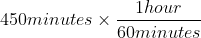You can see here that "minutes" is in both numerator and denominator, allowing you to cancel those units.  And it dictates that to get your answer you'll divide 450 by 60.  That reduces as a fraction to 45 divided by 6, which comes out to 7.5.

### Example Question #2 : Solving Word Problems With One Unit Conversion

Tiffany wants to purchase an 18-foot length of rope to hang a tire swing. When she arrives at the store to buy the rope, all of the lengths are quoted in inches. How many inches should she purchase to have exactly 18 feet of rope?

184

144

216

1.5

216

Explanation:

When converting between units, it can be helpful to use dimensional analysis to set up your equation. You know that there are 12 inches in 1 foot, and that you need to convert 18 feet to a number of inches. If you then structure your math so that the units cancel via division, you can ensure that you're making the right "do I multiply or do I divide by 12" decision: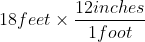Helps you determine that you need to multiply, because then the "feet" units cancel leaving you with the units you want, which is inches.  This means you multiply 18 by 12, which gives you 216 inches.

### Example Question #1 : Solving Word Problems With One Unit Conversion

Kendrick purchased 8 gallons of water for a camping trip, and plans to pour all of the water into insulated flasks that measure 1 quart each to keep the water cool. How many flasks will it take to contain all 8 gallons? (1 gallon = 4 quarts)

32

2

64

16

32

Explanation:

A great way to ensure you make the proper "do I multiply or divide?" decision on any conversion problem is to use dimensional analysis, which means to set up the units in your calculation so that the units you don't want in your answer cancel, and the unit(s) you do want in your answer remain. Here you're given gallons and asked to convert to quarts, so you want to set up the math so that gallons cancels. That means you'll use: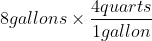This preserves the ratio of 4 quarts to every 1 gallon, and allows the unit "gallons" to cancel via division, so that you're left with quarts. That also means that you multiply 8 by 4 to get the answer, 32.

### Example Question #4 : Solving Word Problems With One Unit Conversion

To prepare for a bicycle race, Celeste wants to ride her bicycle for 20 miles. The app she is using to track her distance only cites distance in kilometers. Using the conversion that 1 kilometer = 0.62 miles, which of the following is closest to the exact number of kilometers she should ride?

21

32

15

39

32

Explanation:

When you're working with conversion problems, it is helpful to use dimensional analysis to make sure that you're making the proper "do I multiply or divide by this conversion" decision. Here you're given a number of miles (she wants to ride 20 miles) and you need to get to kilometers. So you can structure your math with miles in the denominator of the fraction, and that means that the units "miles" will cancel leaving you with just "kilometers":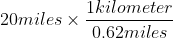As you can see, this tells you to divide by 0.62, and when you've cancelled the unit "miles" you're left with just "kilometers." Note, also, that the question asks for an estimate ("which of the following is closest") so you can safely divide by just 0.6, or 3/5, to get to 33.33, and the only answer that is close is 32.

### Example Question #5 : Solving Word Problems With One Unit Conversion

Brionna uses a fitness app to track the number of steps she takes each day. For the month of February, she took a total of 320,000 steps. She estimates that 2,500 of her steps equates to one mile. Approximately how many miles did she walk in February?

144

115

128

100

128

Explanation:

When you're working with conversion problems, it is helpful to use dimensional analysis to ensure that you are properly applying the conversion (i.e. "should I multiply or divide?"). Here you're given a number of steps and you need to convert to miles using the conversion 2,500 steps = 1 mile. You can then set up your math so that the unit "steps" cancels and you're left with just "miles."  That would mean: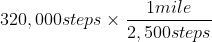As you can see, steps will cancel due to division, meaning that you'll divide 320,000 by 2,500 and be left with the proper unit miles.  To do this math by hand, you should also see that you can factor out 100 from both 320,000 and 2,500, leaving you with the problem 3200 divided by 25.  This leads you to the correct answer, 128.

### Example Question #2 : Solving Word Problems With One Unit Conversion

Caitlyn's goal for the upcoming track and field season is to extend her long jump personal best by 10 inches to break the school record. By approximately how many centimeters will she need to extend her personal best long jump? (1 inch = 2.54 centimeters)

25

54

4

15

25

Explanation:

Whenever you're workin with unit conversions, it is a good idea to use dimensional analysis to structure your math to help you choose the right operation (multiply vs. divide). This means that you'll set up your equation to cancel the units that you don't want in your answer, and therefore you'll be left with the proper units. Here you're given inches and asked to convert to centimeters, so you'll set up the math with inches in the denominator so that the units cancel: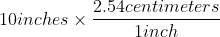This means that you'll multiply 10 by 2.54, and the inch units will cancel ensuring that you're properly converting to centimeters. 10 times 2.54 is 25.4, which rounds to 25.

### Example Question #7 : Solving Word Problems With One Unit Conversion

Ajay plans to adopt a rescue dog from Europe, where the dogs' weights are measured in kilograms. He chooses a dog that weighs 25 kilograms; approximately how many pounds does that dog weigh? (1 kilogram = 2.2 pounds)

11

25

55

42

55

Explanation:

Whenever you're working with unit conversions, it is a good idea to use dimensional analysis to ensure that you are setting up your calculation - specifically the choice of whether you multiply or divide by the conversion ratio - properly. This means that you'll set up the math so that the units you're given cancel, leaving you with the units you need to arrive at in the end. Here you're given kilograms and want to get to pounds, so you'll multiply by a conversion with kilograms in the denominator so that kilograms cancel, leaving you with pounds: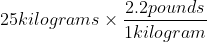This means that you multiply 25 by 2.2, and that kilograms cancel and the only unit left is the one you want, pounds.  25 times 2.2 is 55, giving you the correct answer.

### Example Question #8 : Solving Word Problems With One Unit Conversion

Peter is cooking a large batch of pancakes for a family breakfast. The recipe calls for 16 pints of buttermilk, but the store sells buttermilk in quarts. How many quarts does he need to buy to satisfy the recipe? (1 quart = 2 pints)

4

32

8

16

8

Explanation:

Whenever you're working with unit conversions, it is a good idea to use dimensional analysis to structure your math properly - specifically as it pertains to the question of whether you should multiply or divide by the conversion ratio provided. That means you'll set up the math so that the units you don't want cancel, leaving you with the units you do. Here you're given pints and asked to arrive at quarts, so you'll set up your math such that pints is in the denominator of the conversion ratio so that it cancels. That means that your math will look like: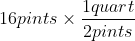This means you'll divide 16 by 2 to get your answer, and since pints cancels via division, you know that you've converted properly to quarts.  16 divided by 2 is 8, making 8 the correct answer.

### Example Question #9 : Solving Word Problems With One Unit Conversion

Aiden is purchasing a red carpet for a gala event. He wants carpet that is 18 feet long, but the vendor quotes all prices in inches. How many inches long does the carpet need to be?

48

1.5

144

216

216

Explanation:

Whenever you're working with unit conversions, it is a good idea to use dimensional analysis to ensure that you are structuring your calculation properly, as often the decision of whether to multiply or divide by the conversion ratio can be tricky. That means that you'll set up the math so that the units you don't want in your answer (here that's feet) will cancel, leaving you with the units you do want (here that's inches). Your math here would look like:Feet here will cancel via division, leaving you with inches. And this tells you to multiply 18 by 12 giving you an answer of 216.

### Example Question #10 : Solving Word Problems With One Unit Conversion

Julie is a zookeeper responsible for feeding baby giraffes. Each giraffe should drink 12 quarts of milk per day, but Julie's milk containers measure in pints. How many pints should she feed each giraffe each day? (1 quart = 2 pints)

36

12

6

24

24

Explanation:

Whenever you're facing a unit conversion problem it is a good idea to use dimensional analysis to help you structure the math - whether you should multiply or divide by the provided conversion ratio - properly. That means that you'll set up the math such that the units you don't want in your answer cancel, leaving you with the units you do want. Here you're given quarts but asked to convert to pints, so you'll set up the math so that quarts are in the denominator and cancel, leaving you with pints: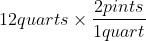This means that quarts cancel leaving you with pints, and tells you that you have to multiply 12 by 2. The correct answer is 24.

### All SAT Mathematics Resources